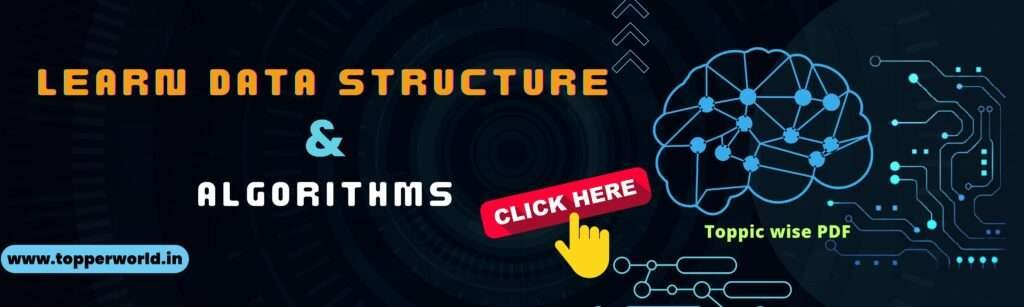### DS Algorithm

Algorithms are the heart and soul of computer science and programming. They are the step-by-step instructions that computers follow to perform tasks, make decisions, and solve problems efficiently. In this article, we’ll demystify algorithms, understand their significance, and explore some fundamental concepts.

At its core, an algorithm is a finite set of well-defined instructions or rules that specify a sequence of operations to solve a particular problem or perform a specific task. Think of it as a recipe in a cookbook: it tells you exactly what ingredients to use and the steps to follow to create a dish.

In the below PDF we discuss about DS Algorithm  in detail in simple language, Hope this will help in better understanding.#### Why Are Algorithms Important ?

Algorithms are the foundation of computer science and have a profound impact on our daily lives. Here’s why they are crucial:

• Efficiency: Algorithms are designed to be efficient. They help computers perform tasks quickly and with minimal resources. For example, search engines use complex algorithms to provide instant search results.
• Problem Solving: Algorithms provide systematic approaches to problem-solving. They help break down complex problems into smaller, manageable steps. Whether it’s optimizing travel routes or processing large datasets, algorithms are at work.
• Reusability: Once created, algorithms can be reused for similar problems. This saves time and effort in software development. For instance, sorting algorithms can be used in various applications without reinventing the wheel.
• Accuracy: Algorithms provide precise and consistent results. Computers follow algorithms without errors, making them reliable for critical tasks like financial calculations and scientific simulations.

#### Elements of an Algorithm :

An algorithm consists of several key elements:

• Input: The data or information that the algorithm operates on. It defines what the problem is and what needs to be processed.
• Output: The result or solution produced by the algorithm. It can be a single value, a set of values, or even actions to be taken.
• Control Structures: These are decision points and loops that guide the flow of the algorithm. They determine what actions to take based on conditions or how many times to repeat certain steps.
• Operations: The specific tasks or actions performed on the input data to transform it into the desired output. These can include mathematical calculations, comparisons, and data manipulation.

#### Related Question

An algorithm is a finite set of well-defined instructions or rules that specify a sequence of operations to solve a particular problem or perform a specific task.

Algorithms are crucial in computer science because they provide efficient solutions to problems, enable automation, and form the basis for software development and data processing.

The key elements of an algorithm include input (data to be processed), output (result produced), control structures (decisions and loops), and operations (tasks performed on the input data).

Control structures guide the flow of an algorithm by making decisions (e.g., if statements) and repeating actions (e.g., loops) based on conditions.

Common algorithm categories include sorting algorithms (e.g., Bubble Sort, Quick Sort), search algorithms (e.g., Binary Search, Linear Search), graph algorithms, dynamic programming, and greedy algorithms.

#### DSA interview Questions

DSA Inetrview Questions Are you

#### Recursion in Data Structure

Recursion in Data Structure Recursion

#### Tree Data Structure

Tree Data Structure Trees are

#### Graph Data Structure

Graph Data Structure Graphs are